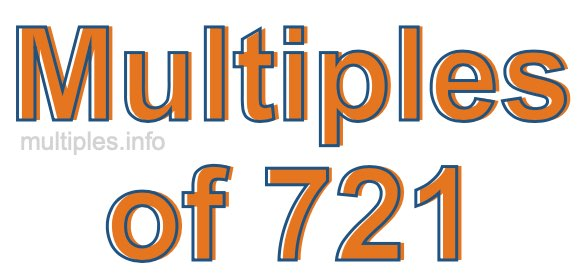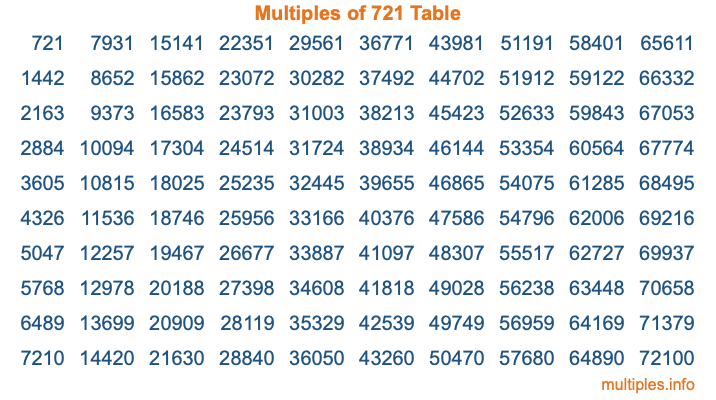Multiples of 721Welcome to the Multiples of 721 page. Here we will first teach you everything you will ever need to know about the multiples of 721, and then give you a study guide summary of everything we taught you to make sure you remember it all. Use this page to look up facts and learn information about the multiples of 721. This page will make you a multiples of seven hundred twenty-one expert!

Definition of Multiples of 721
Multiples of 721 are all the numbers that when divided by 721 equal an integer. Each of the multiples of 721 are called a multiple. A multiple of 721 is created by multiplying 721 by an integer.

Therefore, to create a list of multiples of 721, you start with 1 multiplied by 721, then 2 multiplied by 721, then 3 multiplied by 721, and so on for as long as you want. Thus, the list of the first five multiples of 721 is 721, 1442, 2163, 2884, and 3605. To see a larger list of multiples of 721, see the printable image of Multiples of 721 further down on this page. We also have a category where you can choose any nth multiple of 721.

Multiples of 721 Checker
The Multiples of 721 Checker below checks to see if any number of your choice is a multiple of 721. In other words, it checks to see if there is any number (integer) that when multiplied by 721 will equal your number. To do that, we divide your number by 721. If the the quotient is an integer, then your number is a multiple of 721.

Is  a multiple of 721?

Least Common Multiple of 721 and ...
A Least Common Multiple (LCM) is the lowest multiple that two or more numbers have in common. This is also called the smallest common multiple or lowest common multiple and is useful to know when you are adding our subtracting fractions. Enter one or more numbers below (721 is already entered) to find the LCM.

Check out our LCM Calculator if you need more details about the Least Common Multiple or if you need the LCM for different numbers for adding and subtraction fractions.

nth Multiple of 721
As we stated above, 721 is the first multiple of 721, 1442 is the second multiple of 721, 2163 is the third multiple of 721, and so on. Enter a number below to find the nth multiple of 721.

th multiple of 721

Multiples of 721 vs Factors of 721
721 is a multiple of 721 and a factor of 721, but that is where the similarities end. All postive multiples of 721 are 721 or greater than 721. All positive factors of 721 are 721 or less than 721.

Below is the beginning list of multiples of 721 and the factors of 721 so you can compare:

Multiples of 721: 721, 1442, 2163, 2884, 3605, etc.

Factors of 721: 1, 7, 103, 721

As you can see, the multiples of 721 are all the numbers that you can divide by 721 to get a whole number. The factors of 721, on the other hand, are all the whole numbers that you can multiply by another whole number to get 721.

It's also interesting to note that if a number (x) is a factor of 721, then 721 will also be a multiple of that number (x).

Multiples of 721 vs Divisors of 721
The divisors of 721 are all the integers that 721 can be divided by evenly. Below is a list of the divisors of 721.

Divisors of 721: 1, 7, 103, 721

The interesting thing to note here is that if you take any multiple of 721 and divide it by a divisor of 721, you will see that the quotient is an integer.

Multiples of 721 Table
Below is an image of the first 100 multiples of 721 in a table. The table is in chronological order, column by column. The first column has the first ten multiples of 721, the second column has the next ten multiples of 721, and so on.The Multiples of 721 Table is also referred to as the 721 Times Table or Times Table of 721. You are welcome to print out our table for your studies.

Negative Multiples of 721
Although not often discussed or needed in math, it is worth mentioning that you can make a list of negative multiples of 721 by multiplying 721 by -1, then by -2, then by -3, and so on, to get the following list of negative multiples of 721:

-721, -1442, -2163, -2884, -3605, etc.

Multiples of 721 Summary
Below is a summary of important Multiples of 721 facts that we have discussed on this page. To retain the knowledge on this page, we recommend that you read through the summary and explain to yourself or a study partner why they hold true.

There are an infinite number of multiples of 721.

A multiple of 721 divided by 721 will equal a whole number.

721 divided by a factor of 721 equals a divisor of 721.

The nth multiple of 721 is n times 721.

The largest factor of 721 is equal to the first positive multiple of 721.

721 is a multiple of every factor of 721.

721 is a multiple of 721.

A multiple of 721 divided by a divisor of 721 equals an integer.

721 divided by a divisor of 721 equals a factor of 721.

Any integer times 721 will equal a multiple of 721.

Multiples of a Number
Here you can get the multiples of another number, all with the same attention to detail as we did for multiples of 721 on this page.

Multiples of
Multiples of 722
Did you find our page about multiples of seven hundred twenty-one educational? Do you want more knowledge? Check out the multiples of the next number on our list!